# Numbers - math word problems

#### Number of problems found: 1462

• Chocolates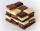In the market we have 3 kinds of chocolates. How many ways can we buy 14 chocolates?
• The difference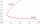The difference of two numbers is 1375. If their exact quotient is 12. Find the two numbers
• In the bowlThere are 12 pears in the bowl. Erik ate a third. How many pears remained in the bowl?
• A numberA number increased by 7.9 is 8.3
• 10th termWhat is the 10th term of the Arithmetic Progression if x1=4 and d=5?
• Summands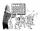Find two summands of the number 42, so that its product is minimized.
• Trigonometric functionsIn the right triangle is: ? Find the value of s and c: ? ?
• Balls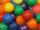I have 19 balls and my friend has 27. About how many has my friend more balls?
• RemainderA is an arbitrary integer that gives remainder 1 in the division with 6. B is an arbitrary integer that gives remainder 2 the division by. What makes remainder in division by 3 product of numbers A x B ?
• Find the 21Find the sum of the six terms of the finite geometric sequence 96, -48, 24, -12
• Expression 6Evaluate expression: -6-2(4-8)-9
• Package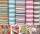The package was 23 meters of textile. The first day sold 12.3 meters. How many meters of textile remained in the package?
• No. of divisorsHow many different divisors have number ??
• What is one third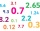What is 1/3 as a decimal? Give your answer rounded to 2 decimal places.
• Page numberingThe book has 88 pages. How many times is the number 4 used for the book numbering?
• Insert 3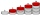Insert five arithmetic progression members between -7 and 3/2.
• Number unknownAdela thought the two-digit number, she added it to its ten times and got 407. What number does she think?
• Division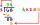Divide by the number 0.2 is the same as multiply by what number?
• Car inflation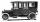About what percentage price of car increased if price rose from €1880 to €2200?
• Dinesh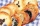Dinesh ate 3 cookies, each with mass 1.45 g. What was the total mass of the cookies that he ate?

Do you have an interesting mathematical word problem that you can't solve it? Submit a math problem, and we can try to solve it.

We will send a solution to your e-mail address. Solved examples are also published here. Please enter the e-mail correctly and check whether you don't have a full mailbox.

Please do not submit problems from current active competitions such as Mathematical Olympiad, correspondence seminars etc...